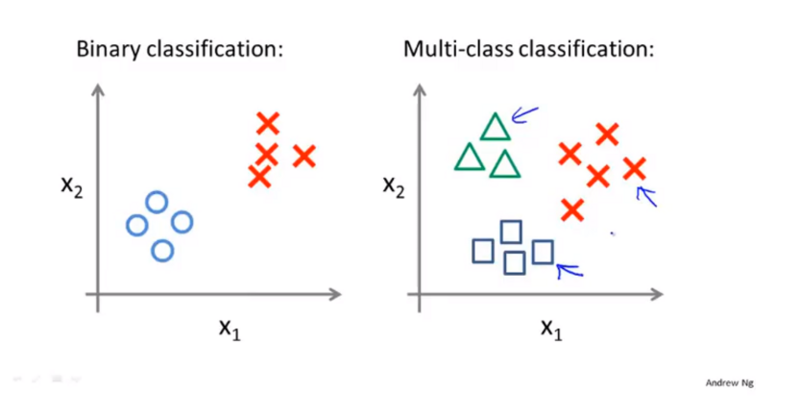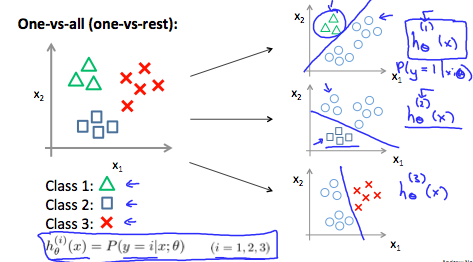## 回归函数

J(\theta) = \frac{1}{m}\sum_{i=1}^{m}\frac{1}{2}(h_\theta(x^(i))-y^(i))^2

Cost(h_\theta(x(i)),y)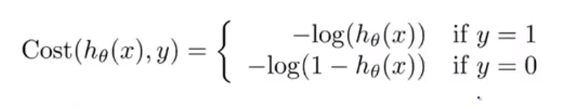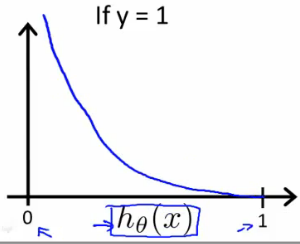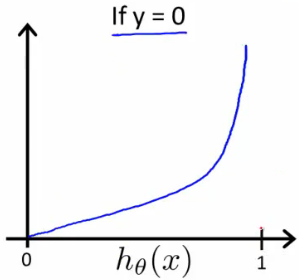Cost(h_\theta(x),y) = -ylog(h_\theta(x)) – (1-y)log(1-h_\theta(x))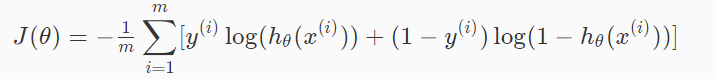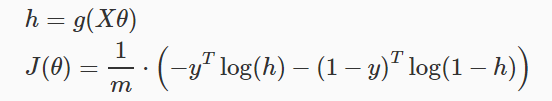## 梯度下降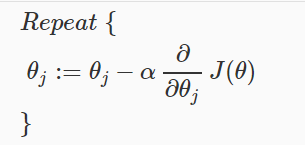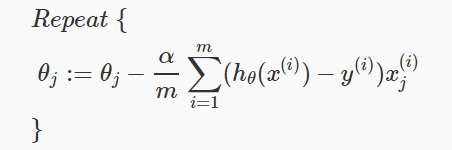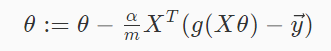## 高级优化

• 不需要手动选择学习速率\alpha
• 往往比梯度下降算法要快一点
• 但相对来说更加的复杂

function [jVal, gradient] = costFunction(theta)
jVal = [...code to compute J(theta)...];
gradient = [...code to compute derivative of J(theta)...];
end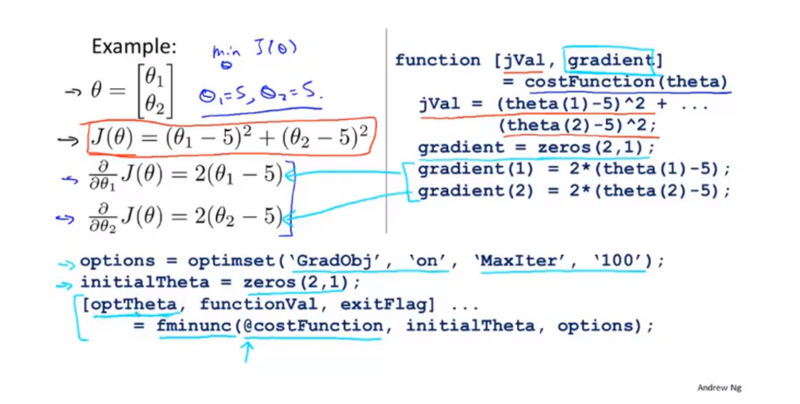## 多类别分类问题